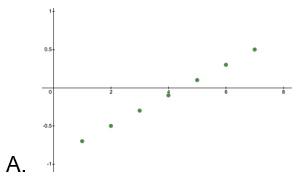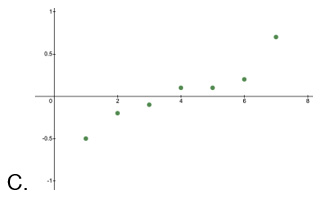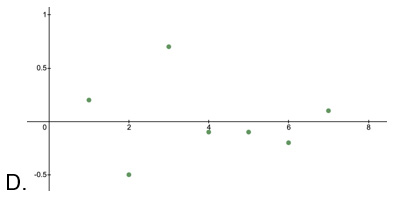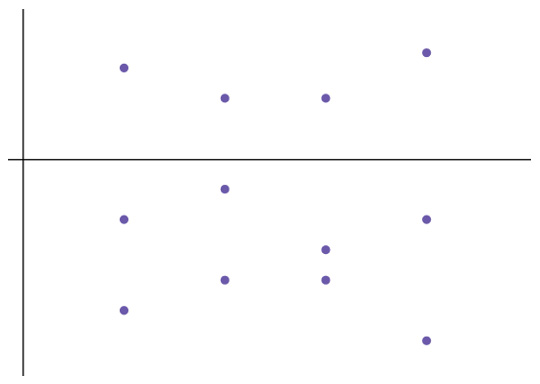# AP Statistics Unit 2 Practice Test: Exploring Two-Variable Data

Test 2 for AP Stats.

Congratulations - you have completed .

You scored %%SCORE%% out of %%TOTAL%%.

Your performance has been rated as %%RATING%%

 Question 1

### From 2010-2020, the correlation coefficient between students’ performance on the NJ graduation exams and the NJ graduation rate is 0.885. Based on this information, which of the following statements are true?

 A Everyone who passed the exam automatically graduates. B There was a strong correlation between passing the exam and graduating. C There was no correlation between the NJ graduation exam and the graduation rate. D A and B
Question 1 Explanation:
The answer B is correct. While the two variables have a strong correlation (correlation coefficient close to 1), this does not mean that one variable caused the other.
 Question 2

### V.  In 3 more weeks, given the same growth rate, the plant will be approximately 3.75 cm tall.

 A Statements I and III B Statements II and IV C Statements II and V D None of the above
Question 2 Explanation:
Linear regression models follow the form $y=a+bx$ where $x$ is the explanatory variable and $y$ is the dependent variable; b is the slope, or the change over time. In this case, the plant was 3 cm tall when first measured (the y-intercept), and grew at an average rate of 0.25 cm per week. In 3 additional weeks, the plant’s predicted growth will be $3+0.25(8) = 3+2 = 5$ cm.
 Question 3

### Which of the following residual plots indicates a linear association between the variables?

 ABCDQuestion 3 Explanation:
Residual plots that indicate a linear association will be roughly scattered above and below $y=0$. D is the only plot that fits this characteristic. The other plots show a pattern that would indicate that nonlinear association is more suitable.
 Question 4

### What can we say about the correlation for that data shown in the scatter plot?### V.  Weak correlation

 A I and III B II and III C I and IV D I and V
Question 4 Explanation:
The graph shows both variables increase together, indicating a positive correlation. The data is clustered fairly tightly along the line, indicating a strong correlation. Answer A is correct.
 Question 5### A student created a least squares regression line, then calculated residuals to determine if it was the most appropriate line for the data. The student got the residual plot shown. What is true about the least squares regression line?

 A The line underestimates the data. B The line overestimates the data. C A non-linear model would be more appropriate. D The least squares regression line is correct.
Question 5 Explanation:
If a linear least squares regression line is the correct line for the data, then the residuals will be scattered above and below the axis in the blot. Because more of the residuals are below the line, but the residuals don’t follow any other pattern, it indicates that a linear model is the best fit, but most of the data points are below the least squares regression line and the line overestimates the data. Answer B is correct.
Once you are finished, click the button below. Any items you have not completed will be marked incorrect.
There are 5 questions to complete.
 ← List →